# Chemistry Assignment Help with Quantum Numbers

## 3.16 Pauli Exclusion Principle

It states that no two electrons in an atom can have the same set of four quantum numbers.

It means that no two electrons in an atom are alike and hence that the total number of electrons in an orbital cannot exceed two.

## 3.17 Quantum Numbers

It is a set of numbers which define the status of an electron inside an atom completely.

The four quantum numbers are

(1) Principal Quantum No. (n)Proposed by Bohr to explain the lines in the atomic spectrum.

(i) the main orbit in which electrons are located.

(ii) Approximate distance of electron cloud from the nucleus.

(iii) energy associated with the orbit.

(iv) Velocity of the electron in the orbit.

Maximum numbers of electrons in nth orbit = 2n2.

It can acquire any value ranging from 1 to ¥, and the corresponding orbits are designated as K, L, M, N ······· shells.

Maximum number of orbitals = n2 in a given orbit

(2) Angular Momentum or Azimuthal Quantum No. (l)

Proposed by Sommerfeld to explain the hyperfine splitting in atomic spectrum

(i)The number of subshells present in a given orbit.

(ii) Relative energies of the subshells.

(iii) Shapes of orbitals.

(iv) Total numbers of orbitals present = (2l + 1)

Total numbers of electrons present = (4l + 2).

(b) For a particular value of n, l can acquire all the values ranging from 0 to (n – 1).

l = 0 s-subshell Spherical

l = 1 p-subshell dumbell

l = 2 d-subshell double dumbell

l = 3 f-subshell complicated

(c) Angular momentum of an orbital =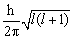(3) Magnetic Quantum No (m)

Proposed by Lande to explain the splitting of lines in a magnetic field.

(i) number of orbitals present in a subshell

(ii) Orientation of orbitals in three dimensional space.

(b) For a particular value of ‘l’ ‘m’ can acquire values from –l to +l including zero

For s-subshell, l = 0, m = 0

For p- subshell, l = 1, m = –l, 0, +1 designated as px, py and pz.

For d- subshell l = 2, m = –2, –1, 0, +1, +2, designated as dxy, dyz, dxz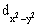and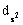For f-subshell l = 3, m = –3, – 2, – 1, 0, 1, +1, +2, +3, which are difficult to represent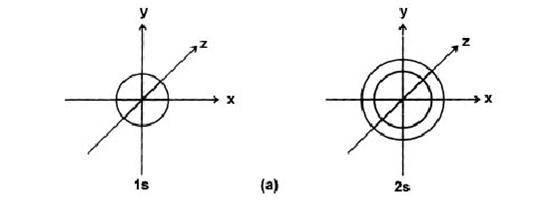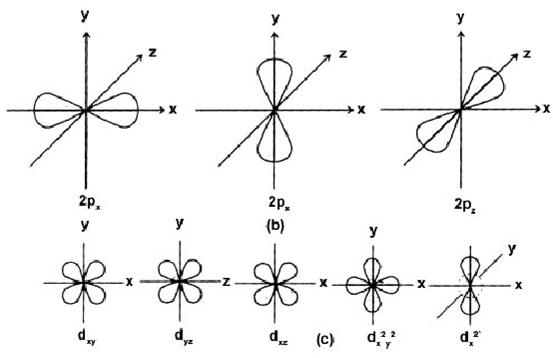(4) Spin Quantum No. (ms)

Proposed by Uhlenbeck & Goudshit, to explain the magnetic properties of substances.

(i) It tells us about the spinning motion of electrons whether the spin is in clockwise or

anticlockwise.

(ii)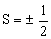(iii) Spin angular momentum =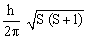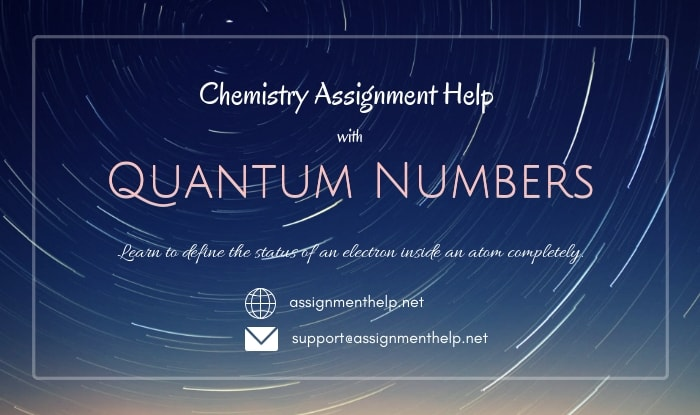### Email Based Assignment Help in Quantum Numbers

Quantum Numbers is a topic in chemistry atomic structure. If you want Assignment Help homework help in Quantum Numbers, assignmenthelp.net online tutors is available for you.